# Trig Graphs

A LevelAQAEdexcelOCR

## Trig Graphs

Back in Trig Basics, we showed you the trig graphs in a pretty simple form. We’ll be looking at a few different transformations of those graphs in this section.

A LevelAQAEdexcelOCR

## A Quick Reminder

Here’s the $\textcolor{blue}{\sin x}$ graph…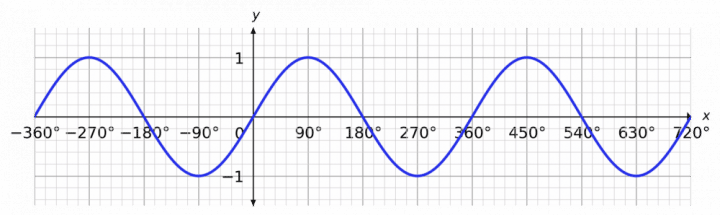and the $\textcolor{limegreen}{\cos x}$ graph…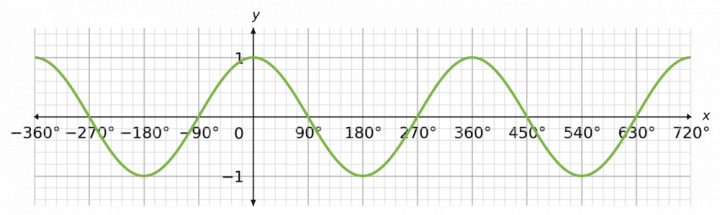… and also the $\textcolor{red}{\tan x}$ graph.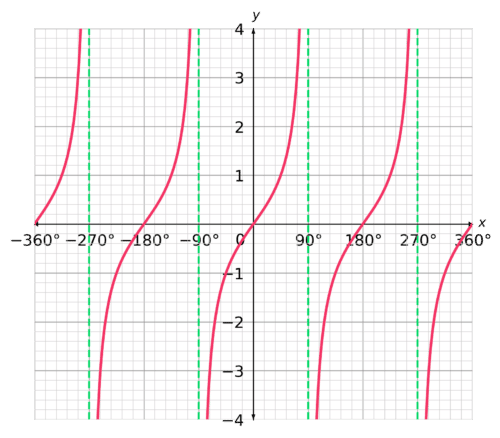So, the $\textcolor{blue}{\sin x}$ and $\textcolor{limegreen}{\cos x}$ graphs have a periodicity of $360°$, while the $\textcolor{red}{\tan x}$ has a periodicity of $180°$.

• $\textcolor{blue}{\sin x} = \sin (x + 360°) = \sin (x - 360°)$
• $\textcolor{limegreen}{\cos x} = \cos (x + 360°) = \cos (x - 360°)$
• $\textcolor{red}{\tan x} = \tan (x + 180°) = \tan (x - 180°)$

Now, it’s time to take a look at some transformations.

A LevelAQAEdexcelOCR

## Vertical Translation

So, below we’ve got a graph of $\textcolor{blue}{y = \sin x}$. Nothing out of the ordinary there.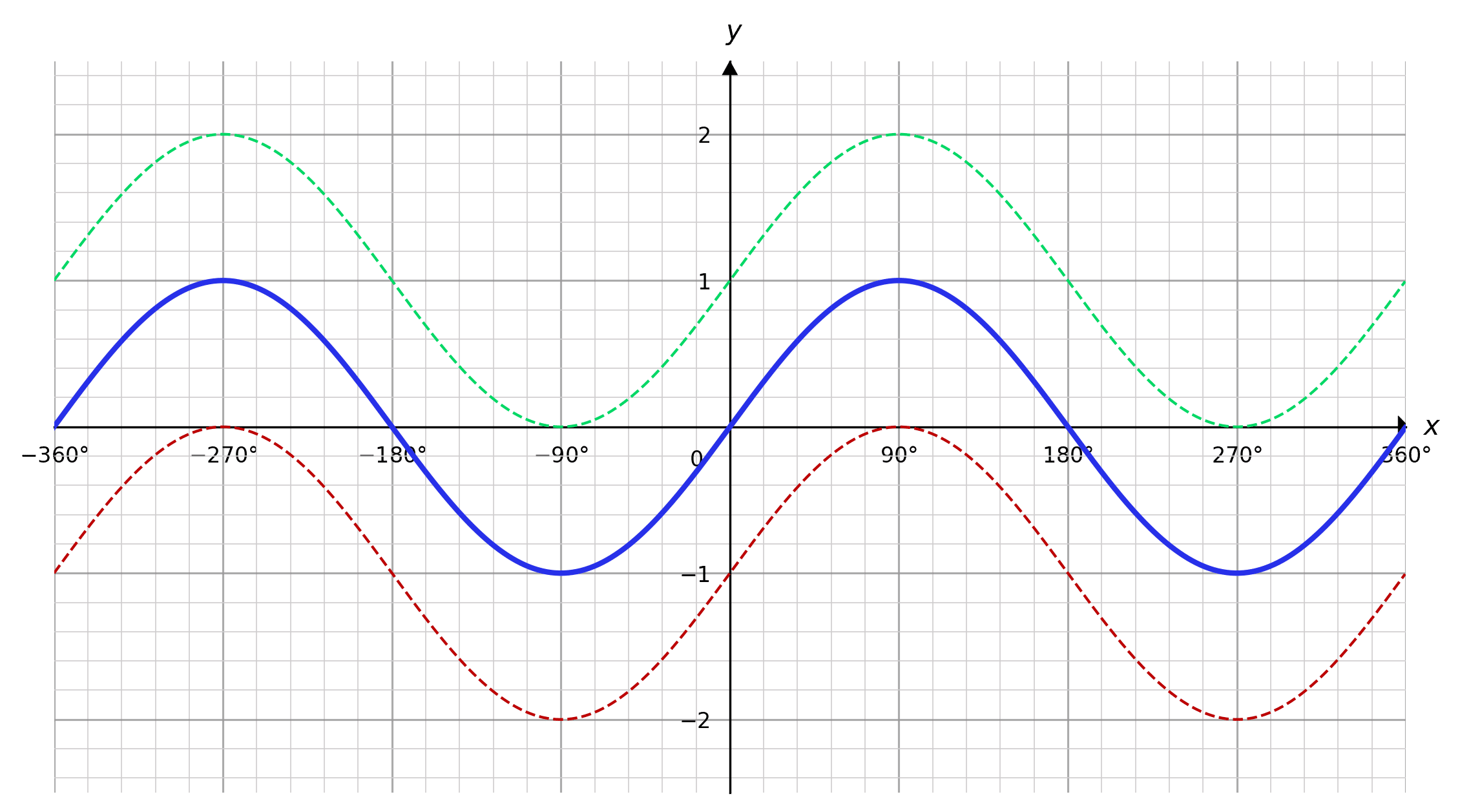We also have two vertical translations, $\textcolor{limegreen}{y = (\sin x) + 1}$ and $\textcolor{red}{y = (\sin x) - 1}$.

A LevelAQAEdexcelOCR

## Horizontal Translation

We also have horizontal translations, where the transformation acts directly on $x$.

Again, we’ll start with $\textcolor{blue}{y = \sin x}$.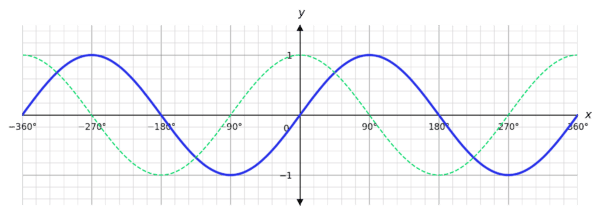Now we’ve got a horizontal translation, $\textcolor{limegreen}{y = \sin (x + 90°)}$.

In short, a transformation $y = \sin (x + c)$ is a translation to the left of $c$. So, if you have $\sin (x - 45°)$, for example, it is a translation $45°$ to the right.

In other words, a transformation of $y = \sin (x + c)$ is a translation of $-c$ along the $x$-axis

A LevelAQAEdexcelOCR

## Vertical Stretching

Now, we’ll introduce vertical stretching to our repertoire.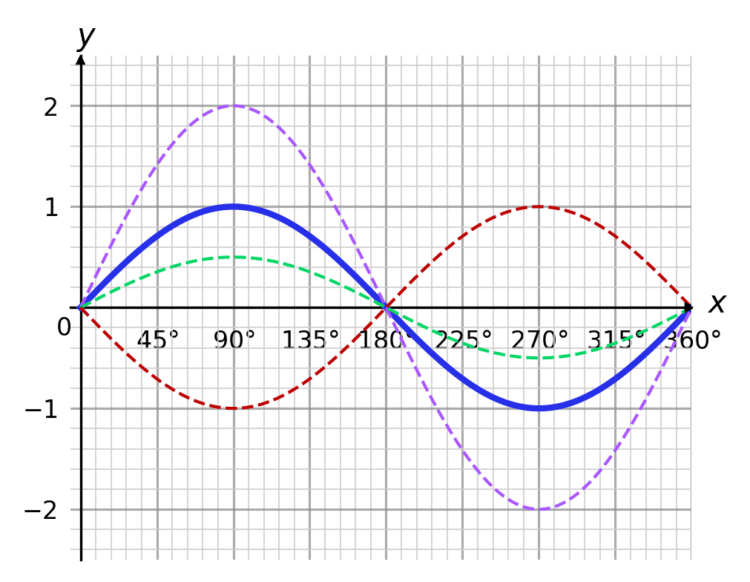We have three transformations of $\textcolor{blue}{y = \sin x}$ here:

• $\textcolor{purple}{y = 2\sin x}$
• $\textcolor{limegreen}{y = \dfrac{1}{2}\sin x}$
• $\textcolor{red}{y = -\sin x}$
A LevelAQAEdexcelOCR

## Horizontal Stretching

We’ll take a look at horizontal stretching now, too.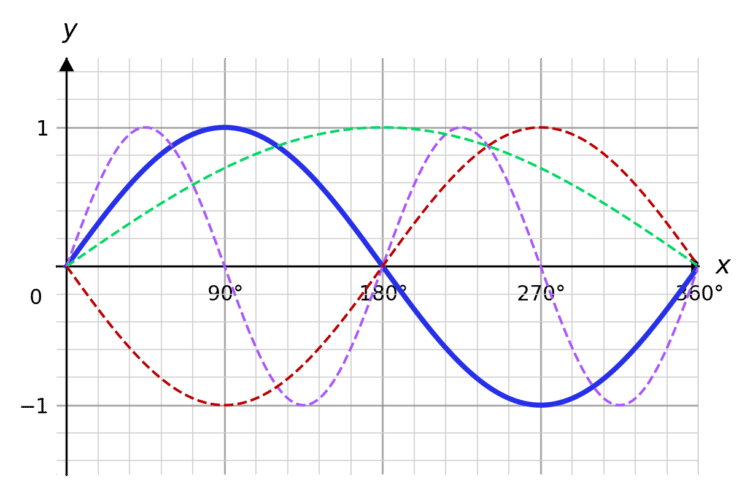We have three transformations of $\textcolor{blue}{y = \sin x}$:

• $\textcolor{purple}{y = \sin (2x)}$
• $\textcolor{limegreen}{y = \sin \left( \dfrac{1}{2}x \right)}$
• $\textcolor{red}{y = \sin (-x)}$
A LevelAQAEdexcelOCR

## A Handy Table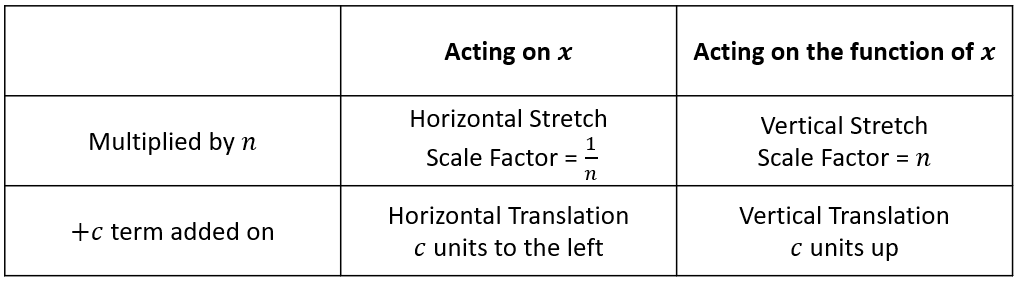A LevelAQAEdexcelOCR

## Trig Graphs Example Questions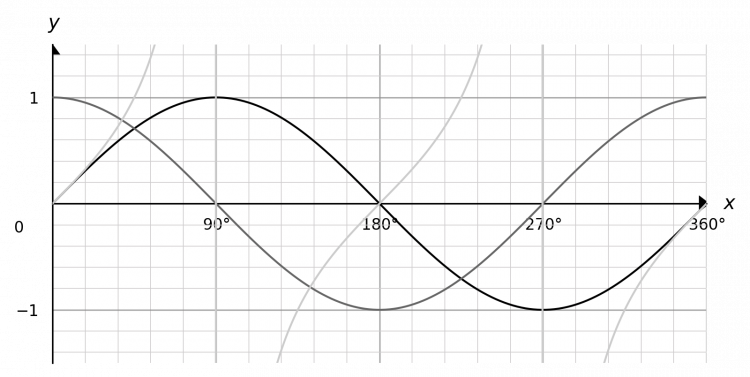$\sin x$ meets the $y$ axis at $y = 0$, and the $x$ axis at $x = 0°, 180°, 360°$.

$\cos x$ meets the $y$ axis at $y = 1$, and the $x$ axis at $x = 90°, 270°$.

$\tan x$ meets the $y$ axis at $y = 0$, and the $x$ axis at $x = 0°, 180°, 360°$. Its asymptotes lie at $x = 90, 270°$.

$y = 2 - 3\sin x$ is a vertical stretch with a scale factor of $-3$, and vertical translation of $+2$. Therefore, its range goes from $-1 \leq y \leq 1 \to -3 \leq y \leq 3 \to -1 \leq y \leq 5$.

$y = \cos \left( 2x - \dfrac{\pi}{2}\right)$ is a horizontal stretch with a scale factor of $\dfrac{1}{2}$, and a horizontal translation of $\dfrac{+\pi}{2}$. There is no vertical transformation, so the range stays at $-1 \leq y \leq 1$.

$y = \tan x$ has an infinite range, so $y = \dfrac{1}{2}\tan x$ also has an infinite range, meaning $-\infty \leq y \leq \infty$.

We need to take the transformations in the order that they affect $x$. So,

1. $\sin x \to \sin \dfrac{x}{2}$ is a horizontal stretch with scale factor $2$
2. $\sin \dfrac{x}{2} \to \sin \left( \dfrac{x}{2} + \dfrac{\pi}{3}\right)$ is a horizontal translation, $\dfrac{\pi}{3}$ units to the left
3. $\sin \left( \dfrac{x}{2} + \dfrac{\pi}{3}\right) \to 3\sin \left( \dfrac{x}{2} + \dfrac{\pi}{3}\right)$ is a vertical stretch with scale factor $3$

Note:

We could alternatively have Step 3 at the beginning, but Steps 1 and 2 must be in the order they are in.

A Level

A Level

A Level

## You May Also Like...### MME Learning Portal

Online exams, practice questions and revision videos for every GCSE level 9-1 topic! No fees, no trial period, just totally free access to the UK’s best GCSE maths revision platform.

£0.00